Thank you for visiting nature.com. You are using a browser version with limited support for CSS. To obtain the best experience, we recommend you use a more up to date browser (or turn off compatibility mode in Internet Explorer). In the meantime, to ensure continued support, we are displaying the site without styles and JavaScript.

# Isolation by distance in a continuous population: reconciliation between spatial autocorrelation analysis and population genetics models

## Abstract

Analysis of the spatial genetic structure within continuous populations in their natural habitat can reveal acting evolutionary processes. Spatial autocorrelation statistics are often used for this purpose, but their relationships with population genetics models have not been thoroughly established. Moreover, it has been argued that the dependency of these statistics on variation in mutation rates among loci strongly limits their interest for inferential purposes. In the context of an isolation by distance process, we describe relationships between a descriptor of the spatial genetic structure used in empirical studies, Moran’s I statistic and population genetics parameters. In particular, we point out that, when Moran’s I statistic is used to describe correlation in allele frequencies at the individual level, it provides an estimator of Wright’s coefficient of relationship. We also show that the latter parameter, as a descriptor of genetic structure, is not influenced by selfing rate or ploidy level. Under specific finite population models, numerical simulations show that values of Moran’s I statistic can be predicted from analytical theory. These simulations are also used to estimate the time taken to approach a structure at equilibrium. Finally, we discuss the conditions under which spatial autocorrelation statistics are little influenced by variation in mutation rates, so that they could be used to estimate gene dispersal parameters.

## Introduction

Isolation by distance, in the context of population genetics, is the process by which geographically restricted gene flow generates a genetic structure, because random genetic drift is occurring locally. It is an important phenomenon to consider whenever the genetic structure or the evolutionary trends of natural populations are to be analysed spatially. Isolation by distance occurs in subdivided populations, when subpopulations exchange genes at a rate dependent upon the distance, or within a continuously distributed population, when dispersal of gametes and/or zygotes is spatially restricted.

The theoretical analysis of isolation by distance was pioneered by Wright (1946) and Malécot (1948). Malécot analysed how kinship (also termed coancestry) between individuals is related to the distance separating them, and many authors have since used this approach to describe how the genetic structure develops in different models of isolation by distance (e.g. Maruyama, 1971, 1972, 1977; Nagylaki, 1978).

Spatial autocorrelation analysis consists of a set of statistics describing how a variable is autocorrelated through space. Interest in these methods in population genetics was first illustrated by numerical simulations of a population subject to isolation by distance (e.g. Sokal & Wartenberg, 1983; Epperson, 1995). These studies suggested that spatial autocorrelation methods could be used as inferential tools, and an increasing number of experimental studies have used this approach (e.g. Epperson, 1993; Hossaert-McKey et al., 1996; Smouse & Peakall, 1999). However, except for Barbujani (1987), few attempts have been made to establish relationships between spatial autocorrelation statistics and descriptors of the genetic structure used in population genetics theory.

Slatkin & Arter (1991) made several criticisms of the use of spatial autocorrelation methods to study the pattern of genetic structure. One of the major problems they pointed out is that different alleles or loci are likely to be subject to different evolutionary forces, in particular varying mutation rates and selection pressures, causing what they termed parametric variation. Hence, average autocorrelations over alleles or loci are meaningless. Given the high stochastic variation – the difference between one realization of a stochastic process and its expectation – generally observed in simulations, which contributes substantially to the variation among alleles and loci, if autocorrelation coefficients cannot be averaged, the power of the method is indeed considerably reduced (Smouse & Peakall, 1999). Another problem, not limited to spatial autocorrelation methods, is the time needed for a structure to reach an equilibrium state, so that it is usually not known whether an observed genetic structure in a natural population is at equilibrium. In this paper, we tentatively aim to bridge the gap between spatial autocorrelation analysis and population genetics models, and we investigate the limitations of spatial autocorrelation analysis in the context of relatively small continuous populations. We will assume the absence of natural selection because our interest focuses on using genetic markers as neutral indicators of population structure to infer gene flow parameters. We will first point out the relationship between one of the most widely used spatial autocorrelation statistics, Moran’s I, and kinship coefficients described in population genetics models. We then carry out numerical simulations of an artificial population to (i) determine the time needed for the genetic structure to reach its equilibrium state under various conditions; (ii) show that the observed genetic structure described using Moran’s I statistic agrees with theoretical models; and (iii) investigate the effect of various population parameters on the spatial genetic structure. Finally, we relate Moran’s I to the approach developed by Rousset (1997) to estimate gene dispersal.

## Descriptors of the spatial genetic structure

### Kinship coefficients

Since the work of Malécot (1948), the theory of population genetics has made extensive use of the concept of identity-by-descent (IBD) of homologous genes – two genes are identical by descent if they share a common ancestor and no mutation has occurred – to analyse the genetic structure of various population models. The genetic structure can be described in terms of a priori kinship coefficients, θ, which represent probabilities of IBD of pairs of genes sampled appropriately. Usually, a priori kinship coefficients cannot be directly inferred in natural populations, because we cannot assess IBD but only identity-in-state (IIS), so that only correlations for genes are measurable. The correlation between homologous genes i and j can be defined as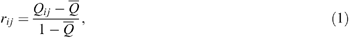where Qij=1 if i and j are IIS, otherwise Qij=0, and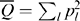with pl being the frequency of allele l in the population (Cockerham & Weir, 1987). In this definition, Q coefficients represent probabilities of IIS. Alternatively, rij can be defined in terms of probabilities of IBD and interpreted as a ‘conditional’ kinship coefficient (Morton, 1973):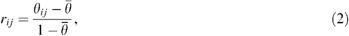where θij is the a priori kinship between genes i and j, and $$\overline{θ}$$ is the a priori kinship between two random genes from the population. The two definitions are equivalent in the infinite allele mutation model (see Rousset, 1996 for other mutation models). If i and j represent pairs of genes within an individual,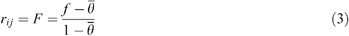is the (conditional) inbreeding coefficient, where f is the probability of IBD of genes within individuals.

In models of isolation by distance within a continuous population, the genetic structure can be described in terms of a priori kinship between pairs of genes according to their spatial position. Then, if θ between the genes of two individuals depends only on the distance, d, separating them, θ( d) provides a complete characterization of the genetic structure. Hence, rij also depends only on the distance between i and j, and r (d) can be inferred from the actual gene frequencies. As a descriptor of the degree of genetic differentiation, the function r(d) in an isolation by distance model is thus analogous to FST, the fixation index, in an island model.

### Spatial autocorrelation methods

Spatial autocorrelation analysis is used to describe how a variable is autocorrelated through space. Hence, it is interesting to relate autocorrelation statistics to population genetics parameters. We consider Moran’s I, one of the most widely used spatial autocorrelation statistics. It is a product–moment coefficient that expresses the correlation of the values of a given variable, defined for a set of locations, between pairs of locations situated at given physical distances apart. It can be computed for each distance class d as (Sokal & Oden, 1978):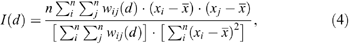where n is the number of localities, xi and xj are the values of the variable at localities i and j, respectively, is the mean value of xi, and wij(d) are weights that equal one if the localities i and j are separated by a distance class d, and equal zero otherwise. Values of Moran’s I plotted against d produce a correlogram that is the function I(d).

To describe a genetic structure, the x variable represents the frequency of a given allele (A), and this frequency can be defined at different levels: a subpopulation, an individual or a gene. At the level of a subpopulation, Barbujani (1987) showed that I(d), the correlation of allele frequencies between pairs of subpopulations separated by a distance d, is an estimator of the ratio r(d)/FST. At the level of a gene (allele frequencies can take only two values: 0 or 1), by definition, Moran’s I statistic gives an estimator of the correlation between genes according to the distance (d), hence an estimator of r(d), the conditional kinship coefficient. In particular, for a pair of genes within an individual (d=0), I(0) is an estimator of F, the (conditional) inbreeding coefficient. To our knowledge, Moran’s I statistic has not been used at the level of a gene in published studies with diploid data. But other estimators of kinship coefficients adapted for multiple alleles and multiple loci have been developed (e.g. Loiselle et al., 1995; Ritland, 1996). Most applications of Moran’s I to describe the genetic structure within a continuous population define allele frequencies at the individual level (in a diploid, allele frequencies equal 0, 0.5 and 1 for genotypes aa, aA and AA, respectively, where a is any allele different from A). Computed in this way, Moran’s I is, by definition, an average estimate per distance class of a parameter known as Wright’s coefficient of relationship, ρ (Cockerham, 1969; Heywood, 1991), i.e. the correlation between average gene frequencies of a pair of individuals. Hence, at the individual level, properties of Moran’s I statistic and its relationship with population genetics models can be deduced from the coefficient of relationship. In the following, we will consider only this application of Moran’s I statistic.

### Coefficient of relationship and kinship coefficient

Wright’s coefficient of relationship, ρij, between any two diploid individuals, i and j, is related to the conditional kinship coefficient by the following equation (Cockerham, 1969):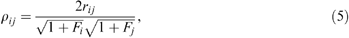where rij is the expected conditional kinship coefficient between a random gene of i and a random gene of j, and Fi is the (conditional) inbreeding coefficient of individual i. More generally, for any ploidy level k (where k is the number of homologous chromosomes assuming polysomic inheritance), and if F is assumed to be constant for all individuals,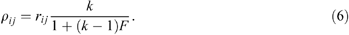Direct estimators of pairwise kinship coefficients have been proposed (Loiselle et al., 1995; Ritland, 1996). Actually, it is easy to demonstrate for a diallelic locus in diploids that the estimator of kinship of Loiselle et al. (1995) is exactly equal to the product of Moran’s I by (1 + F)/2. There is thus no gain in using both Moran’s I and an estimator of kinship coefficients to describe the genetic structure. As a population genetics parameter, ρ can also be defined in terms of a priori kinship coefficients (strictly speaking in the case of an infinite allele model):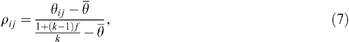where f is the a priori inbreeding coefficient.

### Properties of the coefficient of relationship

As a descriptor of genetic structure, ρ has some interesting properties. Indeed, for a diploid population with selfing allowed and assuming f is constant, Tachida & Yoshimaru (1996) showed that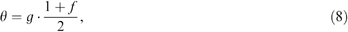where g represents a priori kinship coefficients for a corresponding haploid population (with the same migration and mutation rates). The relationship can be extended to k-ploids (see Ronfort et al., 1998 for a tetraploid):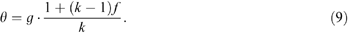Hence, from eqns (7) and (9), the expected value of ρ can be described in terms of the corresponding haploid population:showing that it is independent of the selfing rate, the ploidy level and the presence of double reduction in polyploids (Tachida & Yoshimaru, 1996; Ronfort et al., 1998). Two consequences follow: (i) the coefficient of relationship provides a way of comparing the genetic structure of organisms with different selfing rates or ploidy levels, eliminating the specific effects of ploidy or selfing on the genetic structure, contrary to the kinship coefficient; (ii) estimates of the coefficient of relationship obtained in natural populations can be compared with expected values of haploid population models to infer gene flow parameters. As a descriptor of the genetic structure for an isolation by distance model, ρ(d) is analogous to the parameter ρ defined in Ronfort et al. (1998; eqn 4) for an island model.

Moran’s I is not an ideal estimator of ρ(d), because it has sampling bias (Sokal & Oden, 1978) and gives an estimate for only one allele. Sampling bias can be reduced by adding the value 1/(n − 1) to Moran’s I, where n is the sample size (Sokal & Oden, 1978). Recently, however, an alternative to Moran’s I consisting of a multiallelic multilocus approach to spatial autocorrelation analysis has been developed by Smouse & Peakall (1999).

## Simulation model

Moran’s I statistic is used to describe the genetic structure of a simulated population in order to analyse the rate of approach to equilibrium and to compare the results with the analytical model of Maruyama (1972, 1977). In the simulated model, the organism is diploid and hermaphrodite with nonoverlapping generations. Individuals occupy a habitat in the form of a circle or the surface of a torus and are located in a uniform pattern, at the nodes of a lattice or along an array (lattice model). The genotype of each individual is characterized at one diallelic locus. The initial generation is generated by drawing alternative alleles at random, thus assuming that genotype frequencies follow HardyWeinberg proportions, with initial allele frequencies equal to 0.5. In subsequent generations, new zygotes are produced by drawing an allele per locus from each of two parents from the preceding generation. Each parent is randomly chosen from around the position of the progeny according to a probability law, without distinguishing sexes, derived from a normal distribution (in one or two dimensions) of null average (isotropic dispersal) and of predefined variance, σ2. The actual positions of the individuals being discrete, the model chooses the one located closest to the pointing vector. Self-fertilization occurs at a rate that depends only on the dispersal law. At each generation and for each individual, there is some probability, m, that the individual is replaced by an immigrant with a genotype defined by drawing at random alternative alleles with frequencies equal to 0.5.

In Maruyama’s analytical model, individuals are assumed to occupy random locations, and their movements are independent of each other. However, Felsenstein (1975) demonstrated that these assumptions are actually incompatible with the assumption of a normal distribution of dispersal distances, which would lead to the clumping of individuals, as long as there is no density-dependent selection or migration. In our simulated lattice model, this problem does not occur, because individuals must occupy a discrete set of positions. Another difference from Maruyama’s model is that the so-called ‘stabilizing pressure’ (Imaizumi et al., 1970), m, is not a mutation rate but the rate of immigration of zygotes from a constant source. The use of immigration rather than mutation is justified by the fact that immigration is likely to occur at much higher rates, especially if we focus on a small population or a portion of a larger population, so that realistic values of m cover a larger spectrum.

Values of m that will be used are 0.1, 0.01, 0.001 and 0.0001. Because Wright’s neighbourhood area (NA) has often been used to characterize isolation by distance, we will express the level of localized dispersal in NA equivalents rather than by the axial variance of dispersal distances (σ2). The relationships used for conversion are: NA=4πσ2, in two dimensions; and NA=2πσ, in one dimension, with σ given in lattice units (Wright, 1946). Values of σ used in the simulations were adjusted to obtain NA equal to 10, 30 or 100. It should be noted that the neighbourhood area does not provide a complete description of local differentiation (Slatkin, 1985), and its interpretation as a ‘panmictic unit’ has little theoretical support (Rousset, 1997). Hence, it is used only as a common way to express localized dispersal. Three types of populations were simulated: two toroidal populations with 20 × 20 and 100 × 100 individuals, respectively, and a circular population with 400 individuals. At given time intervals, we estimated the coefficients of relationship for the distance classes 0.5–1.5, 1.5–2.5, …, where distances are given in lattice units, using Moran’s I statistic with sampling bias correction as given above.

## Time to approach equilibrium

To study the number of generations needed for the genetic structure to approach its equilibrium state, starting from a state devoid of structure, we computed Moran’s I(d) values in simulated populations after 1, 2, 4, 8, …, up to 512 generations (or more, if the structure had not yet stabilized), and we defined visually when the shapes of average correlograms did not change any more. We also determined when Moran’s I value for the first distance class, I(1), had reached at least half its equilibrium value. In Fig 1, we can see for a 100 × 100 population with NA=30 and m=0.001 that average correlograms do not change appreciably between 256 and 512 generations, so that it can be assumed that equilibrium has been reached after 256 generations, and half of the equilibrium value of I(1) was reached after eight generations. As noted by Slatkin (1993) for stepping stone models, it clearly appears that spatial structure is limited to short distances for the first generations, and then the correlograms progress outwards with time (Fig 1). The time to approach equilibrium was analysed similarly for 36 simulation sets combining, in a factorial way, the three types of population, the three NA values and the four m values. To obtain reliable average I(d) values, we performed 500 replicates per parameter set for the population of size 20 × 20, 50 replicates for the population of size 100 × 100, and 100 replicates for the circular population 1 × 400.

We observe that the time needed to reach equilibrium increases when the level of dispersal or the immigration rate are smaller (Table 1). For the two-dimensional populations, the time needed to approach equilibrium is longer for the larger population, especially when the immigration rate is small. For populations that bear the same number of individuals but differ in shape, we observe that the one-dimensional population needs substantially more time to approach equilibrium. If the equilibrium state may sometimes be approached only after a relatively long period, the time needed for I(1) to reach at least half its equilibrium value is always quite short (Table 1). Therefore, even if a population is recent and initially devoid of structure, a restricted level of gene dispersal should quickly lead to a detectable genetic structure, at least in the short-distance range. However, if the equilibrium state is needed to make reliable inference on evolutionary processes, it appears that only relatively small populations will reach it in a reasonably short time, unless the rate of gene dispersal or immigration is high.

To relate these simulation results to the theory, it must be emphasized that the observed equilibrium state for correlograms does not involve drift–mutation equilibrium. Hence, what is observed is actually a ‘quasi-equilibrium’ state. Drift–mutation equilibrium is actually assumed in our model because migrants come from a source with constant allele frequencies. The mutation rate, μ, can be neglected, because we assume μ < m. In that condition, drift–mutation equilibrium is approached on a time scale of 1/μ, whereas estimators of genetic differentiation of the type of FST stabilize much more quickly, on a time scale decreasing with higher gene flow and/or lower population size (Maruyama, 1971). As shown above, spatial autocorrelation estimators such as Moran’s I are also of the type of FST; hence, we can expect that they need more time to stabilize with lower m values, lower NA values and/or larger population size, in broad agreement with our results (Table 1).

## Simulation vs. Maruyama’s formulae

We will now compare our estimates of the coefficients of relationship in our simulations, I(d), with the expected values that can be derived from a theoretical model according to Maruyama and adapted for a haploid population. Maruyama has derived formulas for the a priori kinship coefficients in models of populations occupying a circular habitat of circumference L (Maruyama, 1977) or the surface of a toroidal habitat of size L1 × L2 (Maruyama, 1972, 1977), with a population effective density of D. To compute expected kinship, we used the following formulae, where g(x, y) is the a priori kinship coefficient for homologous genes sampled from haploid individuals separated by a distance x along the first axis (L1) and a distance y along the second axis (L2):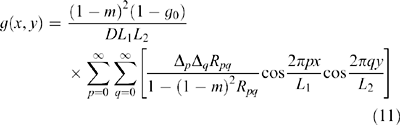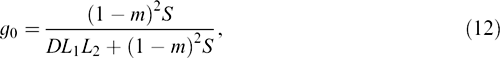where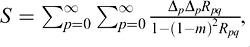with Δ0=1 and Δk=2 when k ≠ 0, and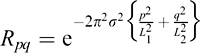if gene dispersal follows a normal distribution of variance σ2.

To obtain the expected values of a priori kinship as a function of the distance d, we set y to zero and let x=d. Actually, for a given distance, the kinship along a diagonal is not exactly equal to that along a vertical or a horizontal line, but we will neglect these differences. For the circular population, we let L2=1. A priori kinship can thus be computed by introducing the following parameters: the population dimensions, L1 and L2; the systematic pressure, m; the variance of gene dispersal distances, σ2; and the population density, D, which is equal to 1.

To convert expected a priori kinship in the haploid population, g(d), into expected coefficients of relationship, ρ(d), we used relationship (10) with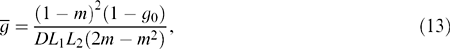as obtained by Maruyama (1972).

The expected coefficients of relationship (i.e. expected Moran’s I values) have been computed for each of the 36 parameter sets for which the time to reach equilibrium had been studied. We obtained a very good agreement between expected and observed Moran’s I values for all parameter sets, and some comparisons can be seen in Fig 2 and Fig 3. This result confirms the validity of our approach to computing expected Moran’s I values from theoretical models expressed in terms of a priori kinship coefficients. It also confirms that mutation, implicit in Maruyama’s model, has the same effect on ρ(d) and r(d) as immigration from a constant source (at least to a very good approximation). Incidentally, it shows that the inconsistencies of Maruyama’s model pointed out by Felsenstein (1975), as mentioned above, are not important when population density is maintained constant, at least within the range of parameters we used.

Theoretical formulae can thus be used to predict the influence of NA and m on the shape of the correlogram. As expected, an increase in gene dispersal (Fig 2), or an increase in gene immigration (Fig 3), both result in correlograms closer to zero values. However, variation in NA and m values does not affect the shape of the correlograms in the same way. In particular, high immigration rates produce correlograms that typically cross the axis at a relatively short distance and, for larger distances, remain almost constant with slightly negative values (Fig 3). In agreement with theoretical expectations (Wright, 1946), it is also clear that one-dimensional populations present a higher level of spatial structure than two-dimensional ones with identical gene flow parameters (Fig 2 and Fig 3).

In Fig 3, we can observe an effect that was deduced analytically by Maruyama (1977): in two-dimensional populations, when m is less than ≈ 1/4N (Nm < 1/4), values of ρ(d) become nearly independent of m (which is not the case for the a priori kinship). As this also holds true if m is a mutation rate, variation in mutation rates among loci can certainly be neglected in two-dimensional continuous populations smaller than about 106 individuals (or 104 for microsatellite loci), that is for most experimental studies that have assessed genetic structure within a population using spatial autocorrelation analysis (e.g. Loiselle et al., 1995; Hossaert-McKey et al., 1996; Smouse & Peakall, 1999). Under these conditions, if neutrality can be assumed, average correlograms over loci can be computed to provide more reliable estimates, for example using the approach of Smouse & Peakall (1999). This is an important conclusion, because it means that one concern of Slatkin & Arter (1991) with regard to spatial autocorrelation methods, about variation in mutation rate, μ, among loci, does not apply for relatively small continuous populations. Discrepancies between our results and those of Slatkin & Arter (1991) can be interpreted as a matter of scale. Slatkin & Arter (1991) simulated a stepping stone model with relatively high mutation rates (μ=2 × 10−4 to 10−3). They did not allow for immigration from an external source, so that mutation was the only stabilizing pressure. As the total population size (N=28 224) in their simulations is higher than 1/(4μ), an effect of the mutation rate is indeed expected. On the contrary, our simulations neglect mutation, assuming μ < m, so that immigration is the main stabilizing pressure and does not vary among loci.

We suggest that the choice of relatively high values of m (in particular m μ) is a reasonable assumption for natural populations in at least two situations. First, when a study focuses on a geographical scale that allows immigration from outside to be larger than μ. This is the case, for instance, in many outcrossing plant populations in which direct estimates of pollen immigration rate are commonly higher than 5% (Hamrick et al., 1995). Secondly, when a substantial fraction of gene dispersal within a population occurs at random. Indeed, in models with m representing a rate of random dispersal throughout the population, hereafter referred to as m (Kimura & Weiss, 1964), the correlograms are strictly identical to models with m being a rate of immigration as long as no allele has come to fixation (results not shown). This situation (m μ) is likely to occur within plant populations given the highly leptokurtic pollen dispersal patterns usually observed (Levin, 1981).

## Application to estimates of gene dispersal

Rousset (1997) presented an approach to the inference of gene flow from F-statistics involving the regression of FST/(1 − FST) estimates for pairs of subpopulations on geographical distance (one-dimensional model) or its logarithm (two-dimensional model). The approach can be extended to a continuous model and provides an estimator of 4π D σ2 (F. Rousset, 1999). According to Rousset (1997), this approach gives good approximations under low mutation rate, μ, and a limited range of distance (e.g. d > σ and d < 0.5σ/2μ in two dimensions). The latter restriction may hinder the study of genetic structure within populations of limited size when the actual σ is large. Moreover, the effect of m or mcan be assimilated to μ, so that even a moderate immigration rate may cause problems.

As shown in the Appendix, the regression approach of Rousset can also be applied to the coefficients of relationship, which gives the following approximate results for k-ploid data: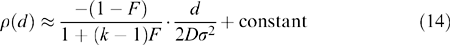in a one-dimensional model, and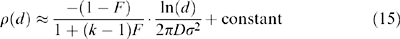in a two-dimensional model. Accordingly, Table 2 shows estimates of gene dispersal, given in NA equivalents, for the 36 simulation sets described previously using the regression of I(d) on d, or ln(d), over all distance classes. As expected, the estimated NA values are closer to their actual values when m is low, and when NA (thus σ) is small relative to the population size.

An alternative to the regression approach, which is less restrictive but more complex to apply, consists of fitting the observed estimates of ρ(d) with their expectations by adjusting the parameters of a theoretical model. In the models we used, two parameters could be adjusted: σ2, the axial variance of gene dispersal distances; and m, the stabilizing pressure, which would be interpreted as the sum of the mutation rate, the immigration rate and the rate of random gene dispersal within the population. However, to demonstrate that reliable inferences can be obtained in this way, it is still necessary to study how robust the theoretical models are to the many deviations from their assumptions that occur in natural populations.

## References

1. Barbujani, G. (1987). Autocorrelation of gene frequencies under isolation by distance. Genetics, 117: 777–782.

2. Cockerham, C. C. (1969). Variance in gene frequencies. Evolution, 23: 72–84.

3. Cockerham, C. C. and Weir, B. S. (1987). Correlations, descent measures: drift with migration and mutation. Proc Natl Acad Sci USA, 84: 8512–8514.

4. Epperson, B. K. (1993). Recent advances in correlation studies of spatial patterns of genetic variation. Evol Biol, 27: 95–155.

5. Epperson, B. K. (1995). Spatial distribution of genotypes under isolation by distance. Genetics, 140: 1431–1440.

6. Felsenstein, J. (1975). A pain in the torus: some difficulties with models of isolation by distance. Am Nat, 109: 359–368.

7. Hamrick, J. L., Godt, M. J. W. and Sherman-broyles, S. L. (1995). Gene flow among plant populations: evidence from genetic markers. In: Hoch, P. C. and Stephenson, A. G. (eds) Experimental and Molecular Approaches to Plant Biosystematics. pp. 215–232. Missouri Botanical Garden, MO.

8. Heywood, J. S. (1991). Spatial analysis of genetic variation in plant populations. Ann Rev Ecol Syst, 22: 335–355.

9. Hossaert-mckey, M., Valero, M., Magda, D., Jarry, M., Cuguen, J. and Vernet, P. (1996). The evolving genetic history of a population of Lathyrus sylvestris: evidence from temporal and spatial genetic structure. Evolution, 50: 1808–1821.

10. Imaizumi, Y., Morton, N. E. and Harris, D. E. (1970). Isolation by distance in artificial populations. Genetics, 66: 569–582.

11. Kimura, M. and Weiss, G. H. (1964). The stepping stone model of population structure and the decrease of genetic correlation with distance. Genetics, 49: 561–576.

12. Levin, D. A. (1981). Dispersal versus gene flow in plants. Ann Mo Bot Gard, 68: 233–253.

13. Loiselle, B. A., Sork, V. L., Nason, J. and Graham, C. (1995). Spatial genetic structure of a tropical understorey shrub, Psychotria officinalis (Rubiaceae). Am J Bot, 82: 1420–1425.

14. Malécot, G. (1948). Les Mathématiques de l’Hérédité. Masson et Cie, Paris.

15. Maruyama, T. (1971). Analysis of population structure. II. Two-dimensional stepping stone models of finite length and other geographically structured populations. Ann Hum Genet, 35: 179–196.

16. Maruyama, T. (1972). Rate of decrease of genetic variability in a two-dimensional continuous population of finite size. Genetics, 70: 639–651.

17. Maruyama, T. (1977). Stochastic Problems in Population Genetics. Springer-Verlag, Berlin.

18. Morton, N. E. (1973). Kinship and population structure. In: Morton, N. E. (ed.) Genetic Structure of Populations pp. 66–69. University of Hawaii Press, Honolulu.

19. Nagylaki, T. (1978). The geographical structure of populations. In: Levin, S. A. (ed.) Studies in Mathematics pp. 588–624. Mathematical Association of America, Washington, DC.

20. Ritland, K. (1996). Estimators for pairwise relatedness and individual inbreeding coefficients. Genet Res, 67: 175–185.

21. Ronfort, J., Jenczewski, E., Bataillon, T. and Rousset, F. (1998). Analysis of population structure in autotetraploid species. Genetics, 150: 921–930.

22. Rousset, F. (1996). Equilibrium values of measures of population subdivision for stepwise mutation processes. Genetics, 142: 1357–1362.

23. Rousset, F. (1997). Genetic differentiation and estimation of gene flow from F-statistics under isolation by distance. Genetics, 145: 1219–1228.

24. Rousset, F. (1999). Genetic differentiation between individuals. J Evol Biol. in press.

25. Slatkin, M. (1985). Gene flow in natural populations. Ann Rev Ecol Syst, 16: 393–430.

26. Slatkin, M. (1993). Isolation by distance in equilibrium and non-equilibrium populations. Evolution, 47: 264–279.

27. Slatkin, M. and Arter, H. E. (1991). Spatial autocorrelation methods in population genetics. Am Nat, 138: 499–517.

28. Smouse, P. E. and Peakall, R. (1999). Spatial autocorrelation analysis of individual multiallele and multilocus genetic structure. Heredity, 82: 561–573.

29. Sokal, R. R. and Oden, N. L. (1978). Spatial autocorrelation in biology. 1. Methodology. Biol J Linn Soc, 10: 199–228.

30. Sokal, R. R. and Wartenberg, D. E. (1983). A test of spatial autocorrelation analysis using an isolation-by-distance model. Genetics, 105: 219–237.

31. Tachida, H. and Yoshimaru, H. (1996). Genetic diversity in partially selfing populations with stepping-stone structure. Heredity, 77: 469–475.

32. Wright, S. (1946). Isolation by distance under diverse systems of mating. Genetics, 31: 39–59.

## Acknowledgements

This work was supported by the Belgian National Fund for Scientific Research (F.N.R.S.) where Olivier Hardy is a research assistant. We would like to thank Dr F. Rousset and Dr M. Schierup, as well as two anonymous reviewers, for their stimulating comments made on a previous version of the manuscript.

## Author information

Authors

### Corresponding author

Correspondence to Olivier J Hardy.

## Appendix

### Appendix

Assuming a low mutation rate in a stepping stone model with k-ploids, it can be shown that the parameters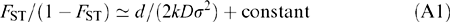in a one-dimensional model, and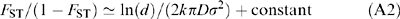in a two-dimensional model, where FST is computed for pairs of populations separated by a distance d, D is an effective population density, and σ2 is the second moment of dispersal distance (Rousset, 1997; Ronfort et al., 1998). An equivalent parameter, a, was developed for a continuous population (F. Rousset, 1999) and defined as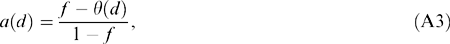where f is the a priori inbreeding coefficient, and θ(d) the expected a priori kinship coefficient between genes separated by a geographical distance, d. Hence, inference of gene dispersal (2) can be obtained from the regression of estimates of a(d) on the geographical distance (one-dimensional population) or its logarithm (two-dimensional population). From eqn (A3), the slope of this regression is equal to −1/(1 − f) times the corresponding slope of θ(d). According to eqn (7), the regression of ρ(d) on geographical distance has a slope equal to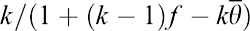times that of θ(d). As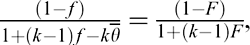eqns (14) and (15) are deduced from eqns (A1) and (A2).

## Rights and permissions

Reprints and Permissions

Hardy, O., Vekemans, X. Isolation by distance in a continuous population: reconciliation between spatial autocorrelation analysis and population genetics models. Heredity 83, 145–154 (1999). https://doi.org/10.1046/j.1365-2540.1999.00558.x

• Accepted:

• Published:

• Issue Date:

### Keywords

• gene flow
• isolation by distance
• population genetics
• simulations
• spatial autocorrelation

• ### High gene flow through pollen partially compensates spatial limited gene flow by seeds for a Neotropical tree in forest conservation and restoration areas

• Patricia Sanae Sujii
• , Evandro Vagner Tambarussi
• , Carolina Grando
• , Ellida de Aguiar Silvestre
• , João Paulo Gomes Viana
• , Pedro H. S. Brancalion

Conservation Genetics (2021)

• ### Using spatial genetics to quantify mosquito dispersal for control programs

• Igor Filipović
• , Hapuarachchige Chanditha Hapuarachchi
• , Wei-Ping Tien
• , Muhammad Aliff Bin Abdul Razak
• , Caleb Lee
• , Cheong Huat Tan
• , Gregor J. Devine
•  & Gordana Rašić

BMC Biology (2020)

• ### Genetic structure and demographic histories of two sympatric Culter species in eastern China

• Ying Xiong
• , Wei Li
• , Jing Yuan
• , Tanglin Zhang
• , Zhongjie Li
• , Wuhan Xiao
•  & Jiashou Liu

Journal of Oceanology and Limnology (2020)

• ### Genetic introgression among differentiated clades is lower among clades exhibiting different parity modes

• J. L. Horreo
• , M. C. Breedveld
• , D. Lindtke
• , B. Heulin
• , Y. Surget-Groba
•  & P. S. Fitze

Heredity (2019)

• ### Geographic distances and ocean currents influence Caribbean Acropora palmata population connectivity in the Lesser Antilles

• Aurélien Japaud
• , Claude Bouchon
• , Hélène Magalon
•  & Cécile Fauvelot

Conservation Genetics (2019)

## Search